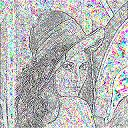Digital Image Processing
Basic effects<- BACK

 Frequencial modification

Low pass filter
Simple 3*3 mask that blur the picture.
Blurring apear beacause of erasing high frequency of the image.

K=9
M=
 1 1 1 1 1 1 1 1 1
Pixel=k*Σ(M(0,0)..M(3,3))

Languages : C & MatLab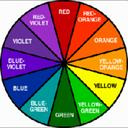Hight pass filter
Simple 3*3 mask that sharp the picture.
Sharping apear beacause of erasing low frequency of the image.

K=9
M=
 -1 -1 -1 -1 4 -1 -1 -1 -1
Pixel=k*Σ(M(0,0)..M(3,3))

Languages : C & MatLab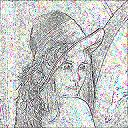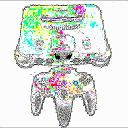Edge detection

Prewitt
Edge detection

K=9
M=
 -2 -1 0 -1 0 1 0 1 2
Pixel=k*Σ(M(0,0)..M(3,3))

Languages : C & MatLab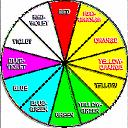Sobel
Edge detection

K=9
M=
 -2 -2 0 -2 0 2 0 2 2
Pixel=k*Σ(M(0,0)..M(3,3))

Languages : C & MatLab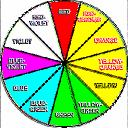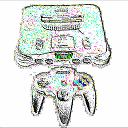Laplacian
Edge detection

K=9
M=
 0 1 0 1 -4 1 0 1 0
Pixel=k*Σ(M(0,0)..M(3,3))

Languages : C & MatLab